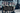# Determine if a string has all unique characters

October 01, 2020## Problem

Implement an algorithm to determine if a string has all the characters as unique.

Lets try to understand the problem first. Always clear out the doubts. Clear about the character set, is it ASCII or what. Before moving on to the solution, declare your input points. Example: you are considering only lower case alphabets.

## Solution using HashSet

A very simple solution can be to use a `HashSet` and just check if you found a character or not. If not, then save it. At any point, if you found a character exists in your `HashSet`, return false.

``````public boolean isUnique(String str) {
Set<Character> set = new HashSet<>();
for (int i=0; i<str.length(); i++) {
if (set.contains(str.charAt(i))) {
return false;
}
}
return true;
}``````

### Complexity

The complexity of this solution would be `O(n)`

## Solution using Array

Clear out from your interviewer the size of your input set. It can be 256 cgaracters or 26 characters. For this solution, lets assume characters to be 256.

We can then take a boolean array of size 256, where each index represents a character position. On finding a character, set it to true.

``````public boolean isUnique2(String str) {
if (str.length() > 256) {
//means at least one character is coming more than once
return false;
}
boolean chars[] = new boolean;

for (int i=0; i<str.length(); i++) {
if (chars[str.charAt(i)]) {
return false;
}
chars[str.charAt(i)] = true;
}
//did not find any duplicate
return true;
}``````

### Complexity

The complexity is also `O(n)`

## Solution using a Bit Vector

For this solution, lets assume we have all lower-case letters. Hence, we have 26 characters in total.

We can take an `Integer` variable which takes `4 bytes = 32 bits`. Which can help us in representing 26 characters and will be out Bit Vector.

``````public boolean isUnique_BitVector(String str) {
if (str.length() >= 26) {
return false;
}
int bit_vector = 0;
for (int i=0; i<str.length(); i++) {
int charVal = str.charAt(i) - 'a';
int shiftedVal = 1 << charVal;

if ((bit_vector & shiftedVal) > 0) {
return false;
}
bit_vector |= shiftedVal;
}
return true;
}``````

In above solution:

• First we are finding the position of the character by doing `val - 'a'`. Example, for character: `b` we will get index=1.
• Then, we will check if there is 1 bit set already or not.
• If its not set, we will set the respective bit.

### Example

``````achc

Assumming last 1 byte of bit vector (8 bits)
Initial bit vector: 0 0 0 0 0 0 0 0

For 'a': charVal=0, 1 << 0 = 1
0 0 0 0 0 0 0 0 & 1 = 0
0 0 0 0 0 0 0 0 | 1 = 0 0 0 0 0 0 0 1

For 'c': charVal=2, 1 << 2 = 1 0 0
(0 0 0 0 0 0 0 0) & (1 0 0) = 0
(0 0 0 0 0 0 0 0) | (1 0 0) = (0 0 0 0 0 1 0 0)

For 'h': charVal=7, 1 << 7 = (1 0 0 0 0 0 0)
(0 0 0 0 0 1 0 0) & (1 0 0 0 0 0 0) = 0
(0 0 0 0 0 1 0 0) | (1 0 0 0 0 0 0) = (0 1 0 0 0 1 0 0)

For 'c': charVal=2, 1 << 2 = (1 0 0)
(0 1 0 0 0 1 0 0) & (1 0 0) = 1
Found > 0, return false``````

### Complexity

Its `O(n)`